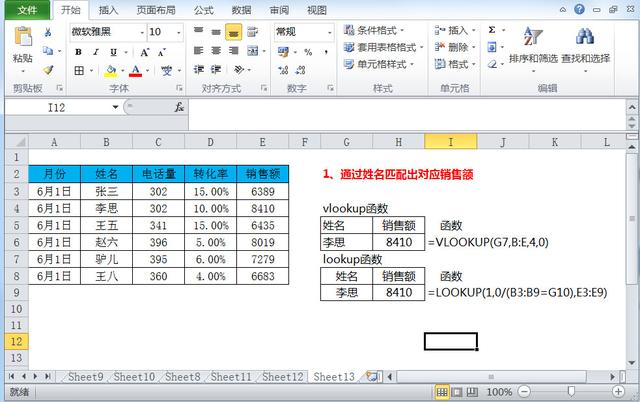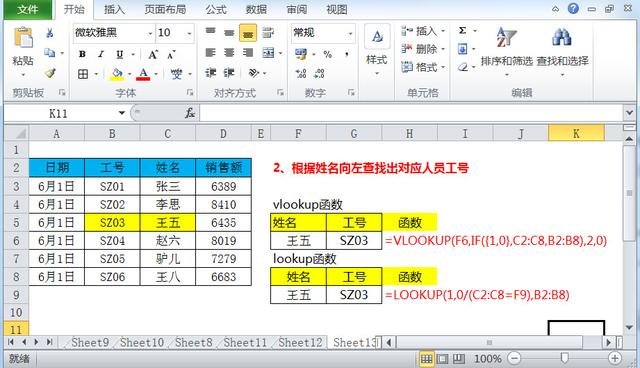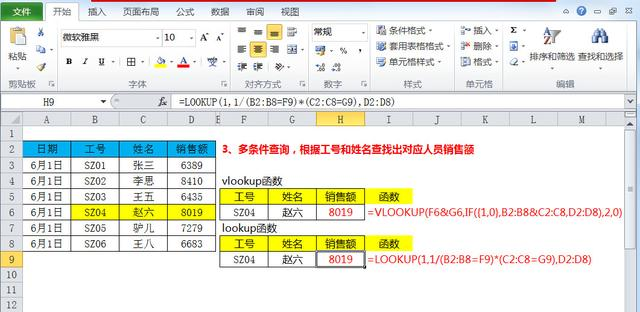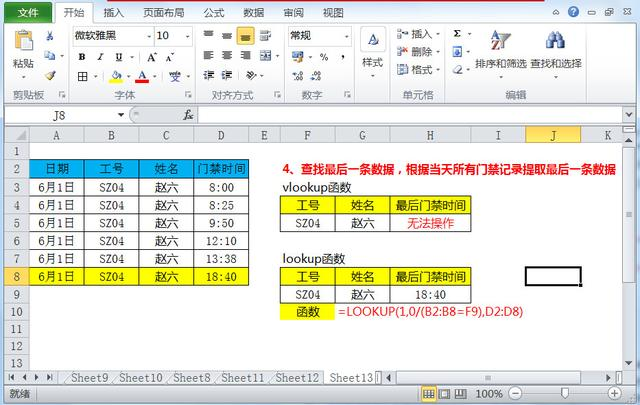Lookup函数可以返回向量或数组中的数值，所以它有两种书写形式。函数形式如下：

（1）向量形式：公式为 = LOOKUP(lookup_value,lookup_vector,result_vector)

（2）数组形式：公式为= LOOKUP(lookup_value,array)，例如：LOOKUP(5.2,{4.2,5,7,9,10})=5。=VLOOKUP(G7,B:E,4,0)

=LOOKUP(1,0/(B3:B9=G10),E3:E9)=VLOOKUP(F6,IF({1,0},C2:C8,B2:B8),2,0)

=LOOKUP(1,0/(C2:C8=F9),B2:B8)={VLOOKUP(F6&G6,IF({1,0},B2:B8&C2:C8,D2:D8),2,0)}

=LOOKUP(1,1/(B2:B8=F9)*(C2:C8=G9),D2:D8)=LOOKUP(1,0/(B2:B8=F9),D2:D8)